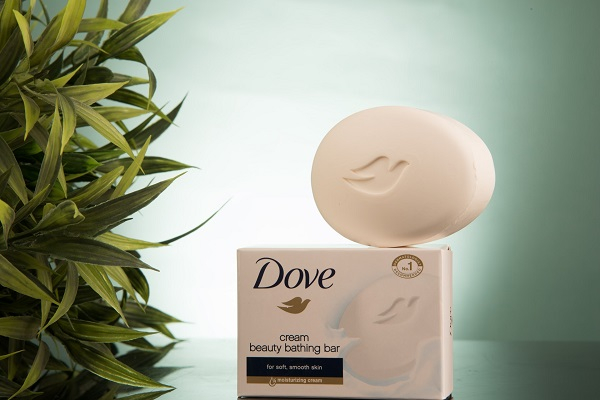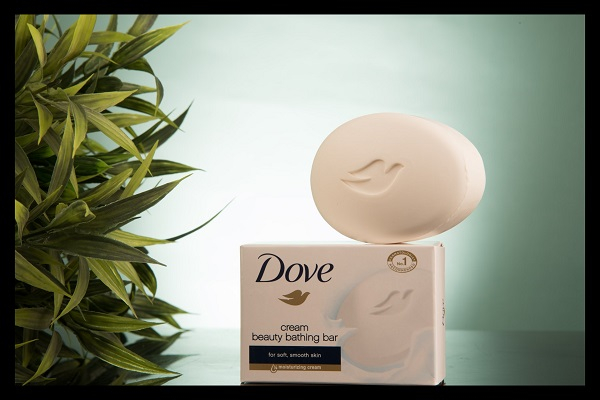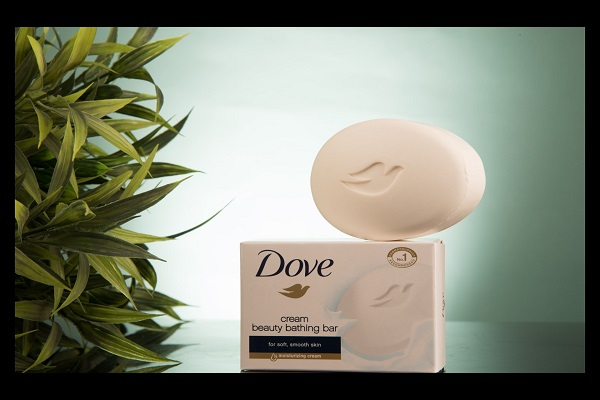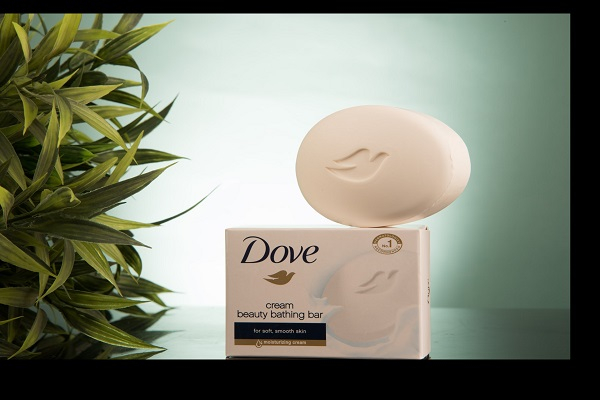# How to pad an image on all sides in PyTorch?

To pad an image on all sides, we can apply Pad() transform provided by the torchvision.transforms module. This module contains many important transformations that can be used to perform different types of manipulations on the image data.

Pad() transformation accepts both PIL and tensor images or a batch of tensor images. A tensor image is a torch Tensor with shape [C, H, W], where C is the number of channels, H is the image height, and W is the image width.

A batch of tensor images is also a torch tensor with shape [B, C, H, W]. B is the number of images in the batch. If the image is neither a PIL image nor a tensor image, then we first convert it to a tensor image and then apply the transform.

## Syntax

torchvision.transforms.Pad(padding)(img)

## Parameters

• Padding – Desired padding size. padding is a sequence like (l, t, r, b), where l, r, t and b are left, top, right, and bottom padding size. The padding may be a sequence of length 2. In this case, left and right padding are same and so are the top and bottom padding. If padding is an int, then the padding will be same on all sides.

## Steps

We could use the following steps to pad an image on all sides −

• Import the required libraries. In all the following examples, the required Python libraries are torch, Pillow, and torchvision. Make sure you have already installed them.

import torch
import torchvision
import torchvision.transforms as transforms
from PIL import Image
• Read the input image. The input image is a PIL image or a torch Tensor.

img = Image.open('dove.jpg')
• Define a transform to pad an image on all sides. Change the padding size according your need.

# padding same for all sides

# to pad 50 -> left/right, 100-> top/bottom

# to pad 0->left, 50->top, 100-> right, 150-> bottom
transform = transforms.Pad((0,50,100,150))
• Apply the above-defined transform on the input image to pad the image on all sides.

img = transform(img)

img.show()

## Input Image

The following image is used as the input in all the examples.## Example 1

# Python program to pad an image on all sides
# import required libraries
import torch
import torchvision.transforms as transforms
from PIL import Image

img = Image.open('dove.jpg')

# compute width and height of image
width, height = img.size

# define a transform to pad an image on all sides

# apply the above transform on the image
img = transform(img)

# resize the image to its original dimension
img = img.resize((width, height))

# dispaly the image
img.show()

## Output

It will produce the following output −Notice that the padding is uniform on all sides in the output image.

## Example 2

# Python program to pad an image on all sides
# import required libraries
import torch
import torchvision.transforms as transforms
from PIL import Image

img = Image.open('dove.jpg')
width, height = img.size

# define a transform to pad an image
# here 50 -> left/right, 100-> top/bottom

# apply the above transforms on the image
img = transform(img)

# resize the image to its original dimension
img = img.resize((width, height))

# dispaly the image
img.show()

## Output

It will produce the following output −Notice that the padding on left and right is same. Similarly, the padding at the top and bottom is uniform.

## Example 3

# Python program to pad an image on all sides
# import required libraries
import torch
import torchvision.transforms as transforms
from PIL import Image

img = Image.open('dove.jpg')
width, height = img.size

# define a transform to pad an image
# here 0->left, 50->top, 100-> right, 150-> bottom
# apply the above transforms on the image
img = transform(img)

# resize the image to its original dimension
img = img.resize((width, height))

# display the image
img.show()

It will produce the following output −Notice that the padding in the output image is different on all sides.